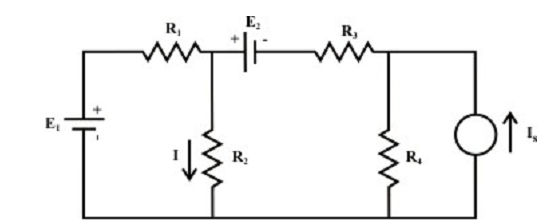## Superposition Theorem Assignment Help

Assignment Help: >> Electrical Engineering - Superposition Theorem

Superposition Theorem

In any linear bilateral network having two or more independent sources (i.e., voltage or current sources or the combination of voltage & current sources), the resultant current or voltage in any branch is the algebraic sum of currents or voltages caused by each independent sources acting all along, with all other independent sources being substituted for the meantime by their respective internal resistances.

Superposition theorem can be described via a simple resistive network as shown in figure below and it has two independent practical voltage sources and one practical current source.One might consider the resistances R1 and R3 are the internal resistances of the voltage sources while the resistance R4 is consider as internal resistance of the current source. The trouble is to establish the response I in the in the resistor R2. The current I can be acquired from

I = I’|due to E1(alone) + I”| due to E2(alone) + I’’’| due to Is(alone)

according to the application of the superposition theorem. It might be observed that each independent source is considered at a time whereas all other sources are turned off. To kill a voltage source means that the voltage source is substituted by its internal resistance (that is R1 and R3; in another words E1 or E2 must be substituted temporarily by a short circuit) while to kill a current source means to substitute the current source by its internal resistance (that is, R4; in another words Is must be substituted temporarily by an open circuit).

Process for using the superposition theorem

Step-1: Maintain one source at a time in the circuit and substitute all other sources with their internal resistances.
Step-2: Compute the output (voltage or current) due to the single source acting only by using the former methods.
Step-3: Repeat steps 1 & 2 for each of the other independent sources.
Step-4: Determine the total contribution by adding up algebraically all the contributions due to an independent sources.

 Application of superposition theorem Compute the current I using superposition theorem Limitations of superposition Theorem